Alternating Current MCQ Level - 2

# Alternating Current MCQ Level - 2

Test Description

## 10 Questions MCQ Test Topic wise Tests for IIT JAM Physics | Alternating Current MCQ Level - 2

Alternating Current MCQ Level - 2 for Physics 2022 is part of Topic wise Tests for IIT JAM Physics preparation. The Alternating Current MCQ Level - 2 questions and answers have been prepared according to the Physics exam syllabus.The Alternating Current MCQ Level - 2 MCQs are made for Physics 2022 Exam. Find important definitions, questions, notes, meanings, examples, exercises, MCQs and online tests for Alternating Current MCQ Level - 2 below.
Solutions of Alternating Current MCQ Level - 2 questions in English are available as part of our Topic wise Tests for IIT JAM Physics for Physics & Alternating Current MCQ Level - 2 solutions in Hindi for Topic wise Tests for IIT JAM Physics course. Download more important topics, notes, lectures and mock test series for Physics Exam by signing up for free. Attempt Alternating Current MCQ Level - 2 | 10 questions in 45 minutes | Mock test for Physics preparation | Free important questions MCQ to study Topic wise Tests for IIT JAM Physics for Physics Exam | Download free PDF with solutions
 1 Crore+ students have signed up on EduRev. Have you?
Alternating Current MCQ Level - 2 - Question 1

### An LCR series circuit with 100Ω resistance is connected to an AC source of 200V and angular frequency 300 radians per second. When only the capacitance is removed, the current lags the voltage by 60°. When only the inductance is removed, the current leads the voltage by 60°. Then the current and power dissipated in LCR circuit are respectively.

Detailed Solution for Alternating Current MCQ Level - 2 - Question 1

When capacitance is removed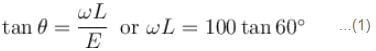when inductance is removed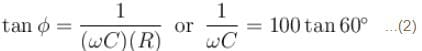From equation (1) and (2)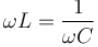So it is condition of resonance.
So  Z = R = 100Ω
I = V/R = 200/100= 2A
Power  P = I2R = 4 ×100 = 400 W
The correct answer is: 2A, 400Watt.

Alternating Current MCQ Level - 2 - Question 2

### In the circuit diagram shown, XC = 100Ω, XL = 200Ω and  R =100Ω. The effective current through the source is :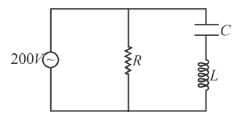Detailed Solution for Alternating Current MCQ Level - 2 - Question 2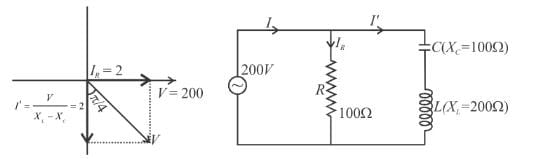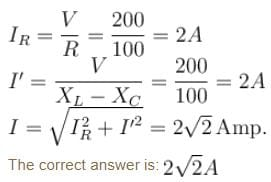Alternating Current MCQ Level - 2 - Question 3

### An ac source of angular frequency ω is fed across a resistor R and a capacitor C in series. The current registered is I. If now the frequency of source is changed to ω/3 (but maintaining the same voltage), the current in the circuit is found to be halved. Then the ratio of reactance to resistance at the original frequency ω is

Detailed Solution for Alternating Current MCQ Level - 2 - Question 3

According to given problem,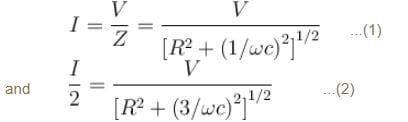Substituting the value of I from equation (1) in (2)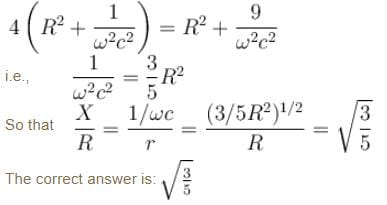Alternating Current MCQ Level - 2 - Question 4

Current in an ac circuit is given by i = 3sin ωt + 4cos ωt, then :

Detailed Solution for Alternating Current MCQ Level - 2 - Question 4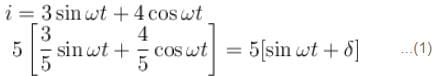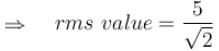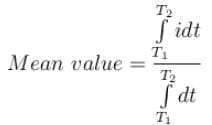∴   Initial value of time is not given hence the mean value will be different for various time intervals.
If voltage applied is  V = Vm sint ωt  then i given by equation (1) indicates that it is ahead by V by  δ   where  0 < δ < 90   which indicates that the circuit contains R and C.

The correct answer is: if voltage applied is V= Vmsin ωt,  then the circuit must be containing resistance and capacitance

Alternating Current MCQ Level - 2 - Question 5

A current source sends a current i = i0 cos ωt. When connected across an unknown load gives a voltage output of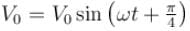across that load. Then voltage across the current source may be brought in phase with the current through it by: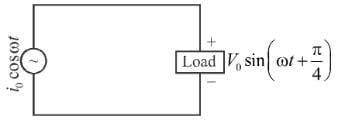Detailed Solution for Alternating Current MCQ Level - 2 - Question 5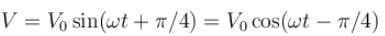Since V lags current, an inductor can bring it in phase with current.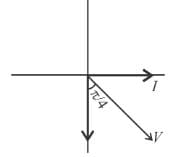The correct answer is: connecting an inductor in parallel with the load

Alternating Current MCQ Level - 2 - Question 6

In series LCR circuit voltage drop across resistance is 8Volt, across inductor is 6Volt and across capacitor is 12Volt. Then:

Detailed Solution for Alternating Current MCQ Level - 2 - Question 6

Since,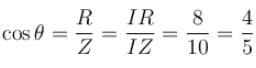(Also  cosθ  can never be greater than 1)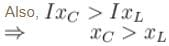∴  Current will be leading
In a LCR circuit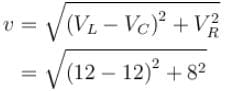V = 10; which is less than voltage drop across capacitor.
The correct answer is: none of these

Alternating Current MCQ Level - 2 - Question 7

For a LCR series circuit with an AC source of angular frequency ω

Detailed Solution for Alternating Current MCQ Level - 2 - Question 7

The circuit will have inductive nature ifAlso if circuit has inductive nature will lag behind voltage.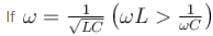the circuit will have resistance nature.
Power factor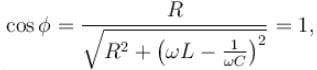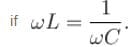Hence  true.

The correct answer is: power factor of circuit will be unity if capacitive reactance equals inductive reactance

Alternating Current MCQ Level - 2 - Question 8

The secondary coil of an ideal step down transformer is delivering 500W power at 12.5A current. If the ratio of turns in the primary to the secondary is 5 : 1, then the current flowing in the primary coil will be :

Detailed Solution for Alternating Current MCQ Level - 2 - Question 8

P = VI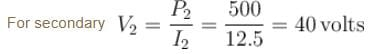For an ideal transformer (100% efficient)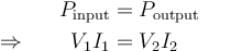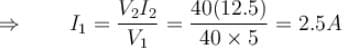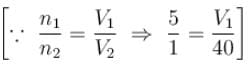The correct answer is: 2.5A

Alternating Current MCQ Level - 2 - Question 9

A bulb is rated at 100V, 100W, it can be treated as a resistor. Find out the inductance of an inductor (called choke coil) that should be connected in series with the bulb to operate the bulb at its rated power with the help of an ac source of 200V and 50Hz.

Detailed Solution for Alternating Current MCQ Level - 2 - Question 9

From the rating of the bulb, the resistance of the bulb can be calculated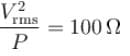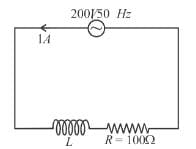For the bulb to be operated at its rated value the rms current through it should be 1A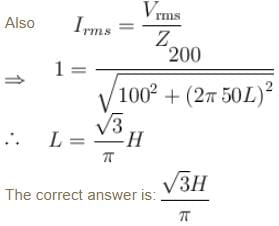Alternating Current MCQ Level - 2 - Question 10

In a black box of unknown elements (L, C or R or any other combination) an AC voltage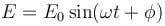is applied and current in the circuit was found to be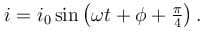Then the unknown elements in the box may be: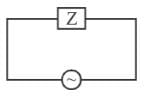Detailed Solution for Alternating Current MCQ Level - 2 - Question 10

If we have all RL and C then I vs, E will be :
To obtain a leading phase difference of
if XL < XC and we use all RL and C in the circuit,
then the resultant graph will be :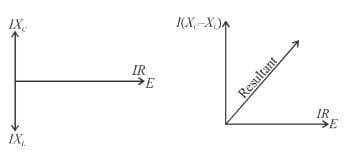which can give a leading phase difference of  π/4.
Similarly if we have only resistance and capacitor then we can obtain a phase difference of  π/4.
(leading) for suitable values of IXC and R. But we cannot obtain a leading phase difference of π/4. If we use only capacitor (phase difference of  π/2), or only (inductor and resistor) (phase difference of  π/2), or only resistor (phase difference of 0).

The correct answer is: either capacitor, resistor and inductor or only capacitor and resistor

## Topic wise Tests for IIT JAM Physics

217 tests
 Use Code STAYHOME200 and get INR 200 additional OFF Use Coupon Code
Information about Alternating Current MCQ Level - 2 Page
In this test you can find the Exam questions for Alternating Current MCQ Level - 2 solved & explained in the simplest way possible. Besides giving Questions and answers for Alternating Current MCQ Level - 2, EduRev gives you an ample number of Online tests for practice

217 tests